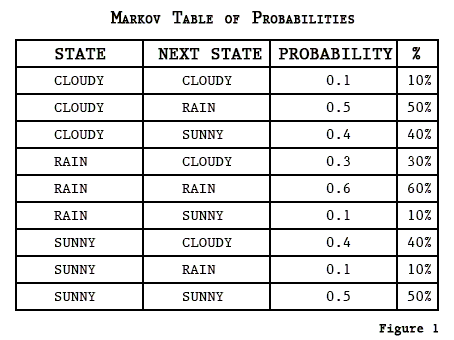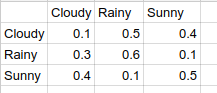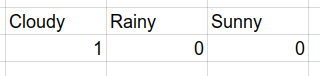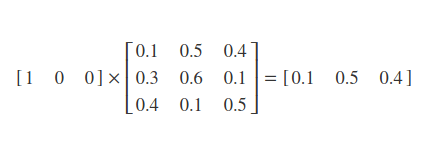# Markow chain explained in simple words

Markow chain is a probabilistic process used to predict the next step based on the probabilities of the existing related states. Its called a chain because the probability of the next step is dependant on the other steps in the group. For example, if the weather is cloudy then its highly likely that it might rain (The next step). If its raining now then the next step would be rainy, sunny or cloudy.

Lets assume the probability of state transitions to be:The above states can be represented in a matrix called the transition matrix as:The Transition Matrix transitions from Row to Column as in the Markov Table. We can do calculations with a Transition Matrix using a State Vector(vector of our current conditions) to give us the probabilities of the next states.

Lets assume that its currently cloudy which gives us as state vector S(1,0,0).To find out the next state in the transition based on the current state, we multiply the state vector with the transition matrix.It will most probably rain than to remain cloudy or sunny.

0 0 vote
Article Rating
Subscribe
Notify of